0

# C语言结构体详解

## 一、结构体的基本概念

``````struct 结构体名
{
结构体成员变量一的声明;
结构体成员变量二的声明;
结构体成员变量三的声明;
......
结构体成员变量四的声明;
};  // 分号不要忘记
``````

### 举个例子

``````struct boy
{
char name;   // 姓名
int  age;        // 年龄
int  height;     // 身高
int  weight;     // 体重
char figure;     // 身材
char face;     // 颜值
}; //记得加分号
``````

## 二、结构体变量

``````  struct boy xiaoqiu1, xiaoqiu2, xiaoqiu3;
``````

## 三、结构体占用的内存情况

### 举个例子

``````#include <stdio.h>
#include <string.h>

// 定义结构体
struct boy
{
char name;     // 姓名
int  age;          // 年龄
int  height;       // 身高
char figure;       // 身材
char face;       // 颜值
};

int main()
{

// 定义结构体变量
struct boy xiaoqiu;

printf("sizeof(struct boy) = %d\n",sizeof(struct boy));
printf("sizeof(xiaoqiu) = %d\n",sizeof(xiaoqiu));

return 0;
}
``````

### 运行效果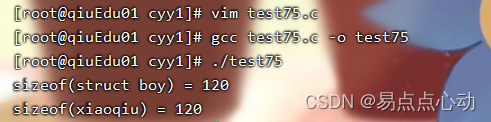C语言提供了结构体成员内存对齐的方法，在定义结构体之前，增加以下代码可以使结构体成员变量之间的内存没有空隙

``````#pragma pack(1) // 在头文件添加
``````

### 再次运行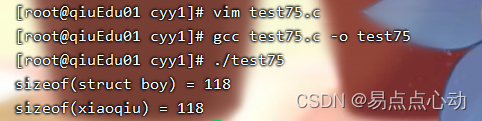## 四、结构体的变量名

``````  struct boy xiaoqiu1;
printf("%d\n",xiaoqiu1);   // 没有意义
printf("%p\n",xiaoqiu1);   // 没有意义，结构体变量名不是结构体变量的地址。
printf("%p\n",&xiaoqiu1);  // 这才是结构体的地址
``````

## 五、结构体初始化

``````  memset(&queen,0,sizeof(struct st_girl));
``````

``````  memset(&queen,0,sizeof(queen));
``````

``````  memset(pst,0,sizeof(struct st_girl));
``````

``````  memset(pst,0,sizeof(pst));
``````

## 五、结构体初始化

``````  memset(&xiaoqiu1,0,sizeof(struct boy));
``````

``````  memset(&xiaoqiu1,0,sizeof(xiaoqiu1));
``````

``````  memset(pst,0,sizeof(struct boy));
``````

``````  memset(pst,0,sizeof(pst));//错误案例
``````

### 举个例子

``````#include<stdio.h>
#include<string.h>
#pragma pack(1)

struct boy
{
char name;
int age;
int height;
char figure;
char face;
};

void func(struct boy *pst)
{
memset(pst,0,sizeof(pst));
memset(pst,0,sizeof(struct boy));

printf("sizeof(pst) = %d\n",sizeof(pst)); // 得到的是指针变量的字节数（8字节）
printf("sizeof(pst) = %d\n",sizeof(struct boy));
}

int main()
{
struct boy xiaoqiu;

memset(&xiaoqiu,0,sizeof(xiaoqiu));
memset(&xiaoqiu,0,sizeof(struct boy));

func(&xiaoqiu);

printf("sizeof(xiaoqiu) = %d\n",sizeof(xiaoqiu));
printf("sizeof(struct boy) = %d\n",sizeof(struct boy));

return 0;
}
``````

### 运行效果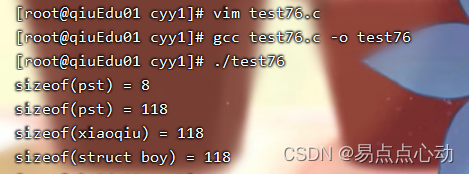## 六、结构体成员的访问

### 举个例子

``````#include<stdio.h>
#include<string.h>
#pragma pack(1)

// 定义一个结构体
struct boy
{
char name;
int age;
int height;
};

int main()
{
// 定义一个结构体变量
struct boy xiaoqiu;

memset(&xiaoqiu,0,sizeof(struct boy));//初始化

strcpy(xiaoqiu.name,"小酋");
xiaoqiu.age = 20;
xiaoqiu.height = 168;

printf("名字： %s\n",xiaoqiu.name);
printf("年龄： %d\n",xiaoqiu.age);
printf("身高： %d\n",xiaoqiu.height);

return 0;
}
``````

### 运行效果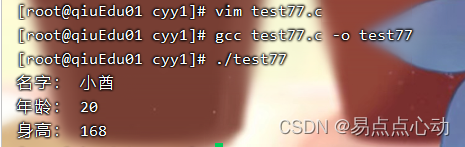## 八、结构体指针

1. 结构体是一种自定义的数据类型，结构体变量是内存变量，有内存地址，也就有结构体指针
2. 在指针章节中我们已经知道，采用不同数据类型的指针指向不同数据类型的变量的地址，这一规则也适用于结构体

``````  struct boy xiaoqiu;
struct boy *pst = &xiaoqiu;
``````

``````  (*pst).memberName
``````

``````  pst->memberName
``````

### 举个例子

``````#include<stdio.h>
#include<string.h>
#pragma pack(1)

// 定义一个结构体
struct boy
{
char name;
int age;
};

int main()
{
// 定义一个结构体变量
struct boy *pst,xiaoqiu;

memset(&xiaoqiu,0,sizeof(struct boy));//初始化

pst = &xiaoqiu;

strcpy(pst->name,"小酋");
pst->age = 20;

printf("名字： %s\n",pst->name);
printf("年龄： %d\n",pst->age);

return 0;
}
``````

### 运行效果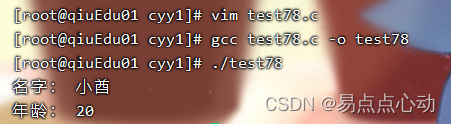## 九、结构体的赋值

``````void *memcpy(void *dest, const void *src, size_t n);
``````

1. src 源内存变量的起始地址
2. dest 目的内存变量的起始地址
3. n 需要复制内容的字节数

### 举个例子

``````#include<stdio.h>
#include<string.h>
#pragma pack(1)

// 定义一个结构体
struct boy
{
char name;
int age;
};

int main()
{
// 定义一个结构体变量
struct boy xiaoqiu1,xiaoqiu2;

memset(&xiaoqiu1,0,sizeof(struct boy));//初始化
memset(&xiaoqiu2,0,sizeof(struct boy));//初始化

strcpy(xiaoqiu1.name,"小酋");
xiaoqiu1.age = 20;

memcpy(&xiaoqiu2,&xiaoqiu1,sizeof(struct boy));

printf("名字：%s 年龄: %d\n",xiaoqiu1.name,xiaoqiu1.age);
printf("名字：%s 年龄: %d\n",xiaoqiu2.name,xiaoqiu2.age);

return 0;
}
``````

### 运行效果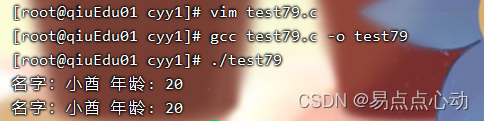## 十、结构体作为函数的参数

### 举个例子

``````#include<stdio.h>
#include<string.h>
#pragma pack(1)

// 定义一个结构体
struct boy
{
char name;
int age;
};

// 给结构体赋值的函数
void setvalue(struct boy *pst)
{

strcpy(pst->name,"小酋");
pst->age = 20;
}

int main()
{
// 定义一个结构体变量
struct boy xiaoqiu1;
memset(&xiaoqiu1,0,sizeof(struct boy));

setvalue(&xiaoqiu1);

printf("名字：%s 年龄: %d\n",xiaoqiu1.name,xiaoqiu1.age);

return 0;
}
``````

### 运行效果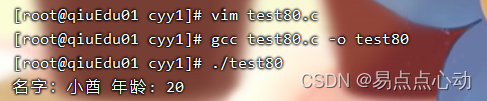### “C语言结构体详解”的评论:

##### 关于作者##### overfit同步小助手